# The graph of y = ax^2 + bx + c

A nonlinear function that can be written on the standard form

$ax^{2}+bx+c,\: \: where\: \: a\neq 0$

is called a quadratic function.

All quadratic functions has a U-shaped graph called a parabola. The parent quadratic function is

$y=x^{2}$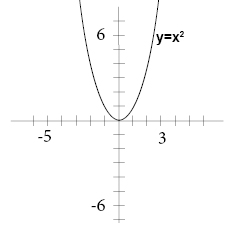The lowest or the highest point on a parabola is called the vertex. The vertex has the x-coordinate

$x=-\frac{b}{2a}$

The y-coordinate of the vertex is the maximum or minimum value of the function.

a > 0                   parabola opens up                    minimum value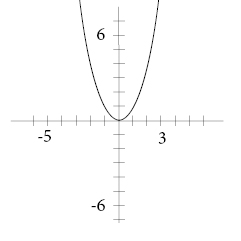a < 0                    parabola opens down              maximum value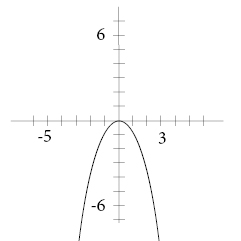A rule of thumb reminds us that when we have a positive symbol before x2 we get a happy expression on the graph and a negative symbol renders a sad expression.

The vertical line that passes through the vertex and divides the parabola in two is called the axis of symmetry. The axis of symmetry has the equation

$x=-\frac{b}{2a}$

The y-intercept of the equation is c.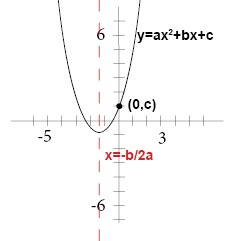When you want to graph a quadratic function you begin by making a table of values for some values of your function and then plot those values in a coordinate plane and draw a smooth curve through the points.

Example

Graph

$x=x^{2}+2x+1$

Make a table of value for some values of x. Use both positive and negative values!

 x y = x2 + 2x + 1 -3 4 -2 1 -1 0 0 1 1 4 2 9 3 16

Graph the points and draw a smooth line through the points and extend it in both directions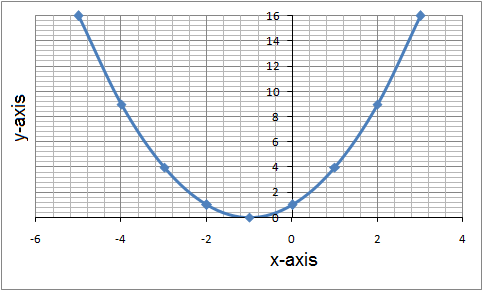Notice that we have a minimum point which was indicated by a positive a value (a = 1). The vertex has the coordinates (-1, 0)  which is what you will get if you use the formula for the x-coordinate of the vertex

$x=-\frac{b}{2a}=-\frac{2}{2\cdot 1}=-1$

and that the line has an y-intercept of (0, 1) which could have been determined from the c-value which is 1.

If you have an absolute value of a that is greater than 1 the parabola will be narrower than the parental quadratic function. And the opposite that if you have a absolute value of a that is less than 1 then the parabola will be wider than the parental quadratic function.

Here you can get a visual of your quadratic equations

## Video lesson

Graph the function

$x^{2} - 3x - 10$